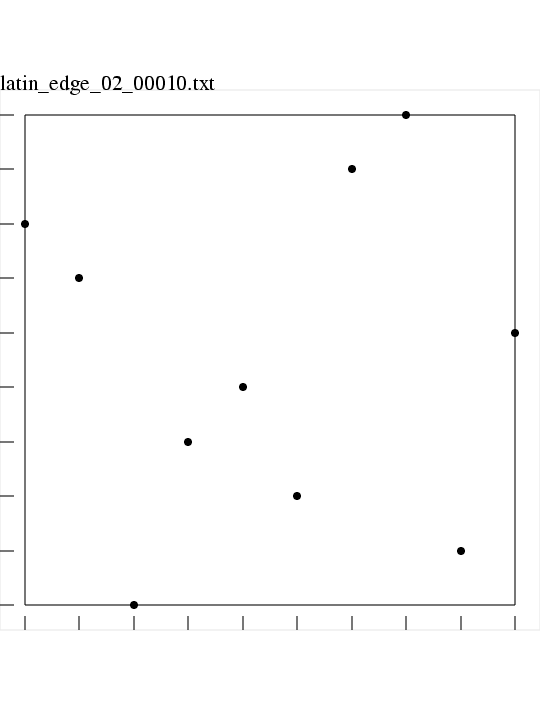# LATIN_EDGE Latin Edge Squares in M dimensions

LATIN_EDGE, a MATLAB library which makes Latin Edge Squares.

A Latin square is a selection of one point from each row and column of a square matrix or table. In M dimensions, the corresponding item is a set of N points, where, in each dimension, there is exactly one point whose coordinates are in a given "column" or range of values. To emphasize the use of higher dimensions, these objects are sometimes called Latin hypersquares.

Once a Latin square has been selected, the center of each subsquare is chosen to yield a Latin Edge Square dataset. This is the same technique that yields a Latin Center Square dataset, with the difference that the mapping from the Latin Square to the unit square is shifted in such a way that the lowest and highest possible coordinate values are 0.0 and 1.0, instead of 1/(2*N) and (2*N-1)/(2*N).

Here is an image of a Latin edge grid with spatial dimension M = 2 and number of points N = 10.### Languages:

LATIN_EDGE is available in a C++ version and a FORTRAN90 version and a MATLAB version.

### Related Data and Programs:

CVT, a MATLAB library which computes elements of a Centroidal Voronoi Tessellation.

FAURE, a MATLAB library which computes elements of a Faure quasirandom sequence.

GRID, a MATLAB library which computes elements of a grid dataset.

HALTON, a MATLAB library which computes elements of a Halton quasirandom sequence.

HAMMERSLEY, a MATLAB library which computes elements of a Hammersley quasirandom sequence.

IHS, a MATLAB library which computes elements of an improved distributed Latin hypercube dataset.

LATIN_CENTER, a MATLAB library which computes elements of a Latin Hypercube dataset, choosing center points.

LATIN_EDGE, a dataset directory which contains a number of examples of datasets created by LATIN_EDGE.

LATIN_RANDOM, a MATLAB library which computes elements of a Latin Hypercube dataset, choosing points at random.

LATTICE_RULE, a MATLAB library which approximates multidimensional integrals using lattice rules.

LCVT, a MATLAB library which computes a latinized Centroidal Voronoi Tessellation.

NIEDERREITER2, a MATLAB library which computes elements of a Niederreiter quasirandom sequence with base 2.

SOBOL, a MATLAB library which computes elements of a Sobol quasirandom sequence.

UNIFORM, a MATLAB library which computes elements of a uniform pseudorandom sequence.

VAN_DER_CORPUT, a MATLAB library which computes elements of a van der Corput quasirandom sequence.

### Reference:

1. Paul Bratley, Bennett Fox, Linus Schrage,
A Guide to Simulation,
Springer Verlag, pages 201-202, 1983.
2. C J Colbourn, J H Dinitz,
CRC Handbook of Combinatorial Design,
CRC, 1996.
3. Bennett Fox,
Algorithm 647:
Implementation and Relative Efficiency of Quasirandom Sequence Generators,
ACM Transactions on Mathematical Software,
Volume 12, Number 4, pages 362-376, 1986.
4. M D McKay, W J Conover, R J Beckman,
A Comparison of Three Methods for Selecting Values of Input Variables in the Analysis of Output From a Computer Code,
Technometrics,
Volume 21, pages 239-245, 1979.
5. Albert Nijenhuis, Herbert Wilf,
Combinatorial Algorithms,